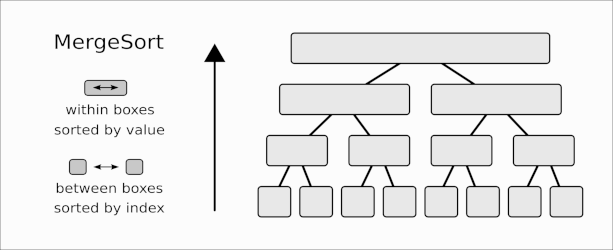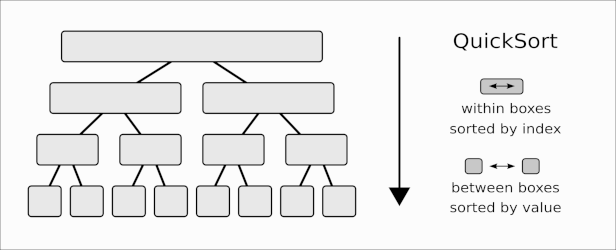# Entries Tagged 'Research' ↓

## A dual view of sorting algorithms

Mergesort and Quicksort are two well-known sorting algorithms, familiar to both researchers and practitioners. Both are typically presented as examples of divide and conquer. Other than this fact, they are usually not seen to be very closely related. Let’s look at Mergesort and Quicksort in a somewhat unusual way that will make them appear as two sides of the same coin.

Suppose we want to sort a list of items $$a_1, a_2, \dots, a_n$$.

In Mergesort, we split the list into two parts: $$L = a_1, \dots, a_{n/2}$$, and $$R = a_{n/2+1}, \dots, a_n$$. We recursively sort $$L$$ and $$R$$, then merge the two sorted lists to obtain the final, sorted output.

How do we sort $$L$$ and $$R$$ recursively? We split them further into equal parts (let’s assume for simplicity that $$n$$ is a power of two, so this always works), until parts consist of single elements, which are already trivially sorted.

So, at the bottom of the recursion tree, we have $$n$$ singleton-lists, consisting of $$a_1, a_2, \dots, a_n$$. Assuming that the splitting has happened “in place”, these items are still stored in their original order, “sorted by their index” $$1,2,\dots,n$$.

See the following illustration.The bottom-most line contains the elements in their original order, one in each “box”. As we come out of the recursion, we merge neighboring “boxes” in pairs, putting their elements in the correct order. So within each box, elements are sorted “by value“.

Observe that neighboring boxes in the same line are sorted “by index“, in the sense that their ordering preserves the original order: indices in any box are smaller than indices in the next box to the right. For example in the second line from the bottom, the first box will contain $$(a_1, a_2)$$ or $$(a_2, a_1)$$, the next box will contain $$(a_3, a_4)$$ or $$(a_4, a_3)$$, etc. the point being that 1 and 2 are both smaller than 3 and 4.

This is Mergesort.

Can we reverse the arrow of time and go from the top towards the bottom? Well, that would be strange, because at the top we have a single box, so this would mean that we start with a single list that is already sorted, which wouldn’t make sense.

But what if by “sorted” we meant “sorted by index“, meaning that the entries are stored as $$(a_1, a_2, \dots, a_n)$$. There is of course no harm in assuming this, as this is indeed how the input is presented. So let’s update the figure.

Instead of bottom-to-top, let’s go top-to-bottom. Let’s also swap “by value” and “by index” throughout.

Now we go from a single box, sorted by index (meaning an unsorted input list) to a list of boxes sorted among them by value (meaning a sorted output list in the bottom). So conceptually, this looks very much like a valid sorting algorithm. What is it? Does it have a name?Yes, it is Quicksort.

Let’s think it through again: we start with a list (sorted “by index”), and we produce from it two lists, sorted between them “by value”. This means that all entries in one are smaller than all entries in the other (this is essentially the partitioning step of Quicksort: we split the elements into those smaller, and those greater or equal than a pivot).

What does it mean that “within a box” the entries are now sorted “by index”? This just means that on both sides of the partitioning we preserve the original ordering of entries. This is not true in every implementation of Quicksort, but it is a technicality we can certainly enforce if we want to. Observe that we assumed that Quicksort splits the list in two equal parts, something we can achieve if we use the median as pivot (in practice of course a random pivot works just fine and is usually faster).

So there you have it. Quicksort and Mergesort are “duals”: you get one from the other by running time backwards, and swapping “by value” and “by index” in the invariants they maintain (or alternatively, swapping “between” and “within”). Yet another way to look at it is that Quicksort does a similar thing as Mergesort, but on the inverse permutation as input.

Surprising? Obvious? Is this view of practical use?

For the latter question, observe that we can extend this view to accommodate unequal splits, or in an extreme case, just splitting off (or merging) one element from/to a longer list. In this view, we obtain (essentially) InsertionSort, respectively SelectionSort, which also appear as duals of each other.

This latter duality extends further, and holds even if we do insertion, resp. selection by fancy data structures, such as self-adjusting binary search trees and adaptive tournaments as heaps. These ideas are explored in a different direction in an article here.

There are also other dualities on ordered sets and data structures (the word “duality” itself is quite overloaded). Exploring dualities often suggests new concepts by symmetry or analogy.

As for the discussion of this post, here is an illustration of both sides.

Illustration of the sorting duality.

## Results on Binary Search Trees

Recently, together with my colleagues Parinya Chalermsook, Mayank Goswami, Kurt Mehlhorn, and Thatchaphol Saranurak, we published three papers about binary search trees. All three are available on ArXiv as preprints. In this post I briefly and informally describe some results and ideas from these papers.

Binary search trees (BST) are perhaps the simplest data structures that are not entirely trivial, and they form the basis of several more advanced data structures and algorithms. BSTs are part of every computer science curriculum, and there is a rich theory describing their properties. Surprisingly, many aspects of BSTs are still not understood, so they are an active field of research.

Searching a single element in a BST is straightforward, and the cost of the search depends on how far the searched element is from the root of the tree. If we search more than one element, we might want to change the tree after each search, to make future searches more efficient. What is the best way to transform the tree after each search? The splay tree of Sleator and Tarjan from 1983 gives one possible such strategy. Splay trees have many nice properties, for example, they are as good as the best static tree for a given sequence (“static” here means not changed between accesses, and I am sweeping some details under the rug here). Sleator and Tarjan conjectured that even more strongly, splay trees are as good as any “dynamic” tree, i.e. any sequence of changes in the tree between accesses. This last statement is the famous dynamic optimality conjecture. If true, this would be quite surprising: splay trees just “react” to each access, without knowing what accesses will come next. The conjecture says that knowing the future doesn’t help much. Even the best strategy for changing the tree (tailored to a given sequence) cannot beat splay trees (again, some details omitted here). The conjecture is wide open. For more information about such questions I recommend the survey of Iacono. Now our recent results.

Splay trees are considered quite mysterious: they execute some local transformations that just “happen to work”. We know that splay trees have many nice properties (including the static optimality mentioned before), but the proofs are a bit unintuitive. What is special about splay trees that gives them these properties? Are there other algorithms that have similar properties?

So we look at a general class of algorithms that preserve some properties of splay trees and relax others. Such an algorithm accesses an element in a BST, then transforms the search path into some tree, such that the accessed element becomes the root. We identify some simple conditions of this transformation that are sufficient to guarantee that the algorithm shares some of the nice properties of splay trees. We look at splay trees in a different way, that makes it obvious that they fulfill these conditions. We also show that some other known algorithms and heuristics fulfill these conditions, which explains why they are efficient. We also identify some new heuristics that fulfill the conditions but have not been studied before. Finally we ask, are our conditions necessary for a BST algorithm to be efficient? In a limited sense, we show that they are, but the results in this direction are not conclusive. See the paper for details.

I mentioned that splay tree is conjectured to be as good as any other BST algorithm, even those that can see into the future, for any possible sequence. However, for most “random” sequences, even the theoretically best BST algorithms are quite inefficient, making the question vacuous in that case. The interesting cases are those sequences that have “some useful structure” that BST algorithms can exploit. So, if we want to show that splay trees (or some other algorithm) are efficient, we need to show that they do well on such “structured” input.

The literature on dynamic optimality describes a number of such “useful structures”. One example is “dynamic finger”: loosely speaking, if successive searches are for values that are close to each other, then search should be efficient. A special case of this is “sequential access”: if we just search the values in increasing order from 1 to n, then search should be really efficient.

In this paper we describe a new kind of structure, that has been studied in depth in mathematics, but not in the context of BSTs. We show that search sequences should be executable efficiently by a BST algorithm if they are free of some fixed pattern. See this page for a description of patterns. This generalizes the “sequential access” mentioned before in a different direction, and it also includes other known structures as special case.
We explore this topic in detail in the paper, giving some general results, and some stronger results for special cases.

Again, studying input with some structure, we look here at a sequence of insert and delete operations into a BST, with the restriction that we can only delete or insert “at the two ends”, that is at values that are the current maximum or minimum of the values in the tree. Such sequences are called deque sequences. It was conjectured a long time ago by Tarjan, that splay trees can execute deque sequences very efficiently (in linear time overall). This is not yet known, but the known results are so close to this, that the difference is only theoretically interesting (which makes it even more interesting :). In this paper (and the previous one) we look at a different algorithm, introduced independently by Lucas and Munro a few years after splay trees, and later extended by Demaine, Harmon, Iacono, Kane, and Pătraşcu. We show that this algorithm (called Greedy BST) is almost linear on deque sequences. This “almost” again hides a small factor that is only of theoretical interest, although a little bit larger than the corresponding factor known for splay trees. The details are in the paper.